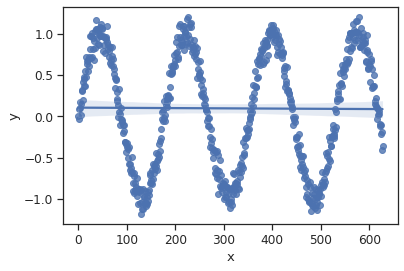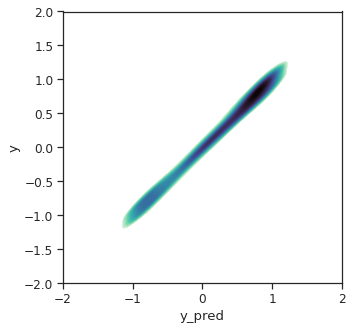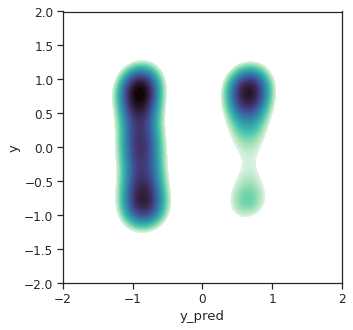First, a quiz.

In the two examples below, is the reported accuracy trustworthy?

1. Janka gets a brilliant idea to predict heart rate just from iPhone movements. She collects time-synchronized data from iPhone movements and heart rate from Apple watch from thousands of consented users. She then splits the data randomly second-by-second into training, validation, and test sets. After she is happy with her model, she reports that she is able to predict heart rate from iPhone movements with a whopping 98% accuracy on the test set!
2. Sam wishes to use satellite imagery to find locations of forests. Sam obtains some training data of sattelite images and human-drawn geolocated maps of forests. Sam then splits the pixels randomly into training, validation, and test sets. After Sam is happy with his model, he reports his test accuracy as 99%!

Is the reported accuracy trustworthy?

NO!

In this article we will learn why they aren't. We will also learn some basic pre-processing principles one can follow to avoid such pitfalls in the future.

Why care about how we split data?¶

Splitting data into training, validation, and test sets, is one of the most standard ways to test model performance in supervised learning settings. Even before we get into the modeling (which receivies almost all of the attention in machine learning), not caring about upstream processes like where is the data coming from and how we split it can have consequences on the quality of predictions.

This is especially important when data has high autocorrelation. Autocorrelation among points simply means that value at a point is similar to values around it. Take temperature for instance. Temperature at any moment is expected to be similar to the temperature in the previous minute. Thus, if we wish to predict temperature, we need to take special care in splitting the data. Specifically, we need to ensure that there is no data leakage between training, validation, and test sets that might exaggerate model performance.

By how much can model performance be exaggerated with information leakage?¶

After reading the above, it is natural to ask, is this an important enough problem for me to care about? Through an example of highly autocorrelated data, we will see that the answer is certainly yes! We will break the example into two parts. First, we will split the data randomly into training and validation sets and achieve a very high accuracy on the validation set. We will then split the data using stratified random sampling, thus reducing information leakage. We will then see how the same model has almost zero accuracy.

Interactive example¶

If you wish to follow this example interactively, you can use this colab notebook.

Let's first import the relevant packages.

In [ ]:
import matplotlib.pyplot as plt
import numpy as np
import pandas as pd
import seaborn as sns
import sklearn.model_selection
import sklearn.linear_model
import sklearn.ensemble

Let's make some synthetic data with high autocorrelation in the response variable

In [ ]:
# number of examples in our data
n = int(100*2*np.pi)
# Seed for reproducebility
np.random.seed(4)
# make one feature (predictor)
x = np.arange(n)
# make one response (variable to predict) which has high autocorrelation. Use a
# sine wave.
y =  np.sin(x/n*7.1*np.pi)+np.random.normal(scale = 0.1, size = n)
# merge them into a dataframe to allow easy manipulation later
df = pd.DataFrame({"x":np.array(x), "y":np.array(y), "y_pred":np.nan})
# visualize the response versus feature
sns.set(style = "ticks", font_scale = 1.1)
sns.regplot(x="x",y="y",data=df)
Out[ ]:
<matplotlib.axes._subplots.AxesSubplot at 0x7f0316d6cc50>Random splitting of data¶

Let's split the data randomly into training and validation sets and see how well the model does.

In [ ]:
# Use a helper to split data randomly into 5 folds. i.e., 4/5ths of the data
# is chosen *randomly* and put into the training set, while the rest is put into
# the validation set.
kf = sklearn.model_selection.KFold(n_splits=5, shuffle=True, random_state=42)
# Use a random forest model with default parameters.
# The hyperparameters of the model are not important for this example because we
# will use the same model twice- once with data split randomly and (later) with
# data split with stratification
reg = sklearn.ensemble.RandomForestRegressor()
# use k-1 folds to train. Predict on the kth fold and store in the dataframe
for fold, (train_index, test_index) in enumerate(kf.split(df)):
reg.fit(df.loc[train_index, "x"].values.reshape(-1, 1), df.loc[train_index, "y"])
df.loc[test_index, "y_pred"] = reg.predict(df.loc[test_index, "x"].values.reshape(-1, 1))
# visualize true y versus predicted y
fig, ax = plt.subplots(figsize = (5,5))
sns.kdeplot(
data=df, x="y_pred", y="y",
fill=True, thresh=0.3, levels=100, cmap="mako_r",ax=ax
)
ax.set_xlim(-2,2)
ax.set_ylim(-2,2)
r2 = sklearn.metrics.r2_score(df.y, df.y_pred)
print(f"[INFO] Coefficient of determination of the model is {r2:0.2f}.")
[INFO] Coefficient of determination of the model is 0.97.Whoa!! We achieved an R$^2$ of 97%! Seems like our model does a fantastic job in modeling the sinusoidal response function.

But ... is the model really able to understand the response function between x and y? Or is it just acting as a nearest neighbour interpolation? In other words, is the model just cheating by memorizing the training data, and outputting the y value of the nearest training example? Let's find out by making it hard for the model to cheat.

Stratified splitting of data¶

Now, rather than splitting the data randomly, we will separate the data into 5 chunks along the x (feature) axis. We will then put 4 chunks into the training data and 1 chunk into the validation set.

Let's see if the model has the same accuracy.

In [ ]:
# How many chunks to split data into?
nbins = 5
df["fold"] = pd.cut(df.x, bins = nbins, labels = range(nbins))

# Split the data into training and validation data based on the chunks.
# Train on 4 chunks, predict on the remaining chunk.
for fold in sorted(df.fold.unique()):
train_index = df.loc[df.fold!=fold].index
test_index = df.loc[df.fold==fold].index
reg.fit(df.loc[train_index, "x"].values.reshape(-1, 1), df.loc[train_index, "y"])
df.loc[test_index, "y_pred"] = reg.predict(df.loc[test_index, "x"].values.reshape(-1, 1))
# Visualize true y versus predicted y.
fig, ax = plt.subplots(figsize = (5,5))
sns.kdeplot(
data=df, x="y_pred", y="y",
fill=True, thresh=0.3, levels=100, cmap="mako_r",ax=ax
)
ax.set_xlim(-2,2)
ax.set_ylim(-2,2)
r2 = sklearn.metrics.r2_score(df.y, df.y_pred)
print(f"[INFO] Coefficient of determination of the model is {r2:0.2f}.")
[INFO] Coefficient of determination of the model is -1.15.Now, we see that our model has below random performance (negative coefficient of determination)! This shows that our initial model was not really using x as an informative predictor for y, rather only to find the nearest x from the training set and spit out the corresponding y. Thus, if we are not careful about autocorrelation in our data, we may have exaggerated model performance.

Worse, we may have erroneously inferred the importance of x, and went on to draw several scientific conclusions. Whereas, our model was using x only to interpolate/memorize the response. This is unfortunately not a made-up example. This paper shows that several papers in the geosciences attempting to predict vegetation biomass (similar to Sam's example in the beginning of this article) are riddled with this problem.

Conclusion¶

Splitting data can have huge consequences. If there is any evidence for data to be autocorrelated, stratified splitting or other techniques for decorrelating the data using signal decomposition can be useful. In the very least visualizing your data before jumping into modeling can be tremendously beneficial. So the next time you meet Sam, Janka, or anyone else who claims to achieve very high modeling performance after randomly splitting their data, you are well equipped to help them come up with better predictive models without exaggerated model performance.